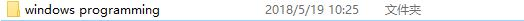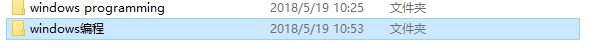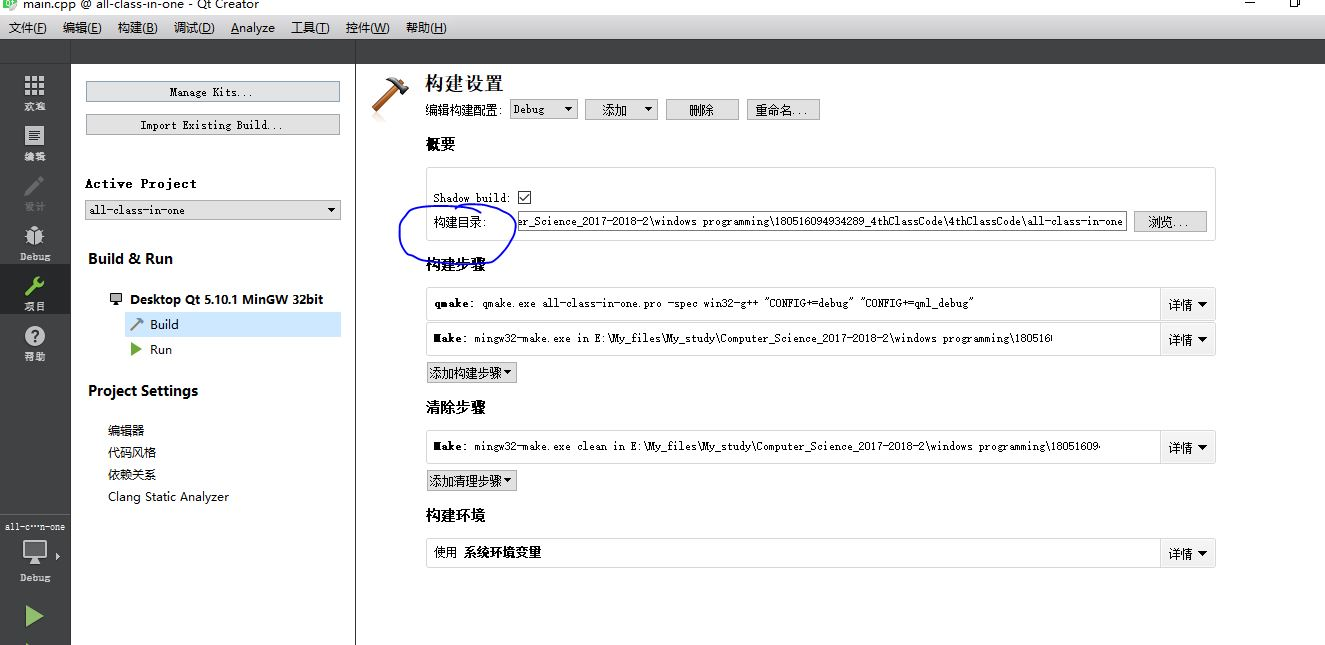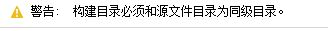## 一、问题描述和解决

Error while building/deploying project all-class-in-one (kit: Desktop Qt 5.10.1 …
When executing step “qmake”## 三、打赏我们

• 我们只对本博客（Luke技术小站（CSDN博客版））的文章中涉及的内容答疑！
• 打赏完全自愿，您可自愿根据问题数下单。
• 您下单后请在您的问题邮件中告知我们订单号，我们将会尽力解答您的问题！（请通过联系作者给我们发邮件！）
• 打赏完全自愿，并非谋利！
• 我们的回复仅供您参考，不保证一定解决您的问题，不承担任何责任！请您理解！
• 如果下面的二维码失效了，请通过此链接完成，谢谢！## HDU1262：寻找素数对

Input

Output

Sample Input

20 30 40

Sample Output

7 13
13 17
17 23

``````#include
#include
using namespace std;

int p = {0};
int tag = {0};

void FindPrime(int a){
int cnt = 0,i;
for (int i = 2; i < a; i++)
{
if (!tag[i])       //tag是标记数组，初始化为0
p[cnt++] = i;    //将数i加入素数数组
for (int j = 0; j < cnt && p[j] * i < a; j++)
{
tag[i*p[j]] = 1;
if (i % p[j] == 0)
break;
}
}
}

void FindResult(int a){
int i,j,num = 0,flag = 0,num1,num2;
for(i = 0; i < 10000; i++){
if(p[i]){
num++;
}
}
for(i = 0; i < num; i++){
for(j = i; j < num; j++){
if((p[i] + p[j]) == a){
if(flag){
if(p[i] > num1){
num1 = p[i];
}
if(p[j] < num2){
num2 = p[j];
}
}
else
{
num1 = p[i];
num2 = p[j];
flag = 1;
}
}
}
}
cout << num1 << " " << num2 << endl;
}

int main(){
int m;
while(cin >> m){
FindPrime(m);
/*for(int i=0;p[i]!=0;i++){
cout << p[i] << "  ";
}*/
FindResult(m);
}
return 0;
}``````

## 2的幂次方表示

1000ms

65536kB

137=27+23+20

2(7)+2(3)+2(0)

3=2+20

2(2(2)+2+2(0))+2(2+2(0))+2(0)

1315=210+28+25+2+1

2(2(2+2(0))+2)+2(2(2+2(0)))+2(2(2)+2(0))+2+2(0)

137

2(2(2)+2+2(0))+2(2+2(0))+2(0)

NOIP1998复赛 普及组 第一题

``````#include
#include
using namespace std;

void Fun(int m);

int main(){
int m;
while(cin >> m){
Fun(m);
cout << endl;
}
return 0;
}

void Fun(int m){
if(m == 2){          //特殊形式单独考虑，递归结束
cout << "2";
return;
}
if(m == 1){         //特殊形式单独考虑，递归结束
cout << "2(0)";
return;
}
int p = 1,n = 0;    //n记录幂次，p是一个数，尽量与m接近
while(p <= m){      //使p与m最接近
p *= 2;
n++;           //最接近时的幂
}
if(m == p/2){        //m正好是一个2次幂，无需带+
cout << "2(";
Fun(n - 1);
cout << ")";
}
else      //m不正好是一个二次幂，需要表示成多项
{
if(p/2==2)      //m是3时，一种特殊情况
{
cout << "2";
cout << "+";
Fun(m-p/2);   //可以直接写为Fun(1)，因为这种情况本身就是m=3时才使用
}
else     //m不是3时的情况
{
cout << "2(";
Fun(n - 1);
cout << ")+";       //加另外的项
Fun(m - p/2);       //将剩余的再递归求解
}
}
}``````

## HDU1002 : A + B Problem II

A + B Problem II

Time Limit: 2000/1000 MS (Java/Others) Memory Limit: 65536/32768 K (Java/Others)
Total Submission(s): 367113 Accepted Submission(s): 71500

Problem Description

I have a very simple problem for you. Given two integers A and B, your job is to calculate the Sum of A + B.

Input

The first line of the input contains an integer T(1<=T<=20) which means the number of test cases. Then T lines follow, each line consists of two positive integers, A and B. Notice that the integers are very large, that means you should not process them by using 32-bit integer. You may assume the length of each integer will not exceed 1000.

Output

For each test case, you should output two lines. The first line is “Case #:”, # means the number of the test case. The second line is the an equation “A + B = Sum”, Sum means the result of A + B. Note there are some spaces int the equation. Output a blank line between two test cases.

Sample Input

2
1 2
112233445566778899 998877665544332211

Sample Output

Case 1:
1 + 2 = 3
Case 2:
112233445566778899 + 998877665544332211 = 1111111111111111110

``````#include
#include
#include
#include
#include
using namespace std;

int m3;
int f = 1;

void Reverse(char *word,int len)   // 反转数字
{
char temp;
int i, j;
for (j = 0, i = len - 1; j < i; --i, ++j) {
temp = word[i];
word[i] = word[j];
word[j] = temp;
}
}

int check(int a[],int num)      //归整
{  int k=0,len=num;
while(a[len-1]==0&&len>1) len--;    //去掉前导0
for(k=0; kif(a[k]>=10)
{
a[k+1]=a[k+1]+ a[k]/10;  a[k]=a[k] % 10;
}
if (a[k]!=0) len=k+1;  //确定数组最终长度
return len;
}

int addition(int m3[], char m1[], int num1, char m2[], int num2)
{
int i,len1,len2,len;
len1=num1;
len2=num2;
len=(len1>=len2)?len1:len2;     //定位数

for(i=0; i<=len; i++) m3[i]=0;   //初始化
for (i=len1; i1; i++) m1[i]='0'; //缺位前导补0
m1[i] = '\0';
for (i=len2; i1; i++)    m2[i]='0';
m2[i] = '\0';
Reverse(m1,len1);
Reverse(m2,len2);
for (i=0; i<=len; i++)
m3[i]=(int)(m1[i]-'0'+m2[i]-'0');   //加法
len=check(m3,len);
return len;
}

int Fun(char a[]){   //确定位数
int i = 0;
for(i = 0; a[i] != '\0'; i++);
return i;  //精确位数
}

int main(){
char num1,num2;
int m,len1,len2,len;
cin >> m;
while(m--){
cin >> num1 >> num2;
len1 = Fun(num1);
len2 = Fun(num2);
if(f != 1){
cout << endl;
}
cout << "Case " << f << ":" << endl;
cout << num1 << " + " << num2 << " = ";
for(int i = len -1; i >= 0; i--){
cout << m3[i];
}
cout << endl ;
f++;
}
return 0;
}``````

## Open Judge2748:全排列

s1 = t1, s2 = t2, …, sp - 1 = tp - 1, sp < tp成立。

abc

abc
acb
bac
bca
cab
cba

``````#include
#include
#include
#include  //在后面使用memset函数和strlen需要包含这个头文件
using namespace std;

const int N=7;
char str[N];
char result[N];
int isVisit[N];
int num = 0;

void PutStr(int n){
if(n == num){
cout << result << endl;
return ;
}
for(int i = 0; i < num; i++){
if(isVisit[i] == 0){
isVisit[i] = 1;
result[n] = str[i];
PutStr(n + 1);  //开始递归
isVisit[i] = 0;
}
}
}

int main(){
memset(result,0,sizeof(result));  //初始化操作
memset(isVisit,0,sizeof(isVisit));
while(cin >> str){
num = strlen(str);
PutStr(0);
cout << endl;
}
return 0;
}``````